#### Determining Rotations for Inertial Explorer and SPAN

Both Inertial Explorer (IE) and SPAN use intrinsic ZXY-order Euler angles to define the rotation between the IMU and output (vehicle) frames, but each has a different approach to how the user should come up with the input angles. The objective is to determine the rotation from the vehicle to the target frame (IMU).

Whenever possible, mount the IMU without rotations (Y forward, X right, Z up). This will help reduce the possibility for errors caused by incorrectly configured rotations.

###### Basic Frames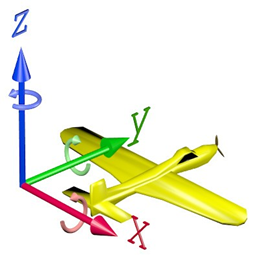Figure 1: Cartesian coordinate system (Vehicle Frame)Figure 2: IMU Frame (Example)

The IMU frame is the objective of the rotations. The rotations are applied to the vehicle frame in the order ZXY until the IMU frame is reached.

The angles are all in a right handed direction. Using your right hand, with your thumb in the direction of the vector, the fingers will curl in the direction of positive rotation.

###### Inertial Explorer

Rotate around the vehicle Z axis until the vehicle Y axis is in the plane that the IMU Y axis would sweep out if rotated about its X axis (this is your Z input angle).

Z angle

Now rotate around the vehicle X axis until the Y axes match (this is your X input angle).

X angle

Now rotate around the vehicle Y axis until both the X and Z axes match (this is your Y input angle).

Y angle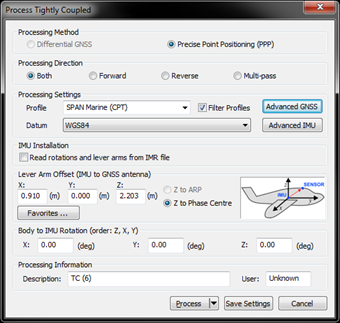###### Example 1 (Inertial Explorer): X = 0 Y = 180 Z = 180

In this example, the IMU (target frame) is mounted with its Y axis backwards, X axis to the right and Z axis down. The vehicle frame is Y axis forward, X axis right and Z axis up.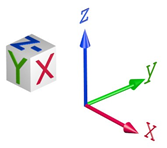Rotate about Z: Starting from the vehicle frame, rotate first about the Z axis (solid blue)

Z = 180 degrees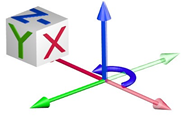Rotate about X: Because the Y axis now matches the IMU there is no need to rotate about X (solid red)

X = 0 degrees

Rotate about Y: Now rotate about the Y axis (solid green) until X and Z match the IMU axes

Y = 180 degrees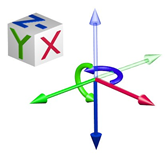Total rotation for input into IE:

X = 0 Y = 180 Z = 180

###### Example 2 (Inertial Explorer): X = 0 Y = 90 Z = -90

In this example, the IMU (target frame) is mounted with its Z axis backwards, Y axis to the right and X axis down. The vehicle frame is Y axis forward, X axis right and Z axis up.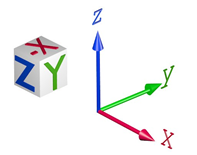Rotate about Z: Starting from the vehicle frame, rotate first about the Z axis (solid blue)

Z = -90 (or 270) degrees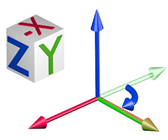Rotate about X: Because the Y axis now matches the IMU there is no need to rotate about X (solid red)

X = 0 degrees

Rotate about Y: Now rotate about the Y axis (solid green) until X and Z match the IMU axes

Y = 90 degrees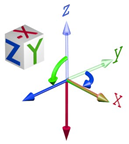Total rotation for input into IE:

X = 0 Y = 90 Z = -90

#### SPAN

When logging from SPAN, for use later in IE, be sure to log the following logs along with the standard post processing profile. If logged, IE will automatically populate and use the rotations from the SPAN file.

LOG IMUTOANTOFFSETSB ONCHANGED LOG VEHICLEBODYROTATIONB ONCHANGED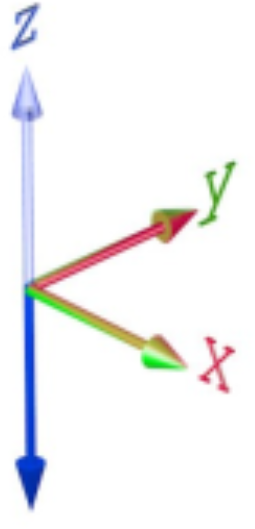Determining what the vehicle to IMU rotation should be: Start with the physical IMU axes. Determine the mapping:

Which way is up? This defines the mapping during a static alignment. Or with kinematic alignments, which orientation did you set using the SETIMUORIENTATION command?

#### IMU Mapping

Table 1: Mapping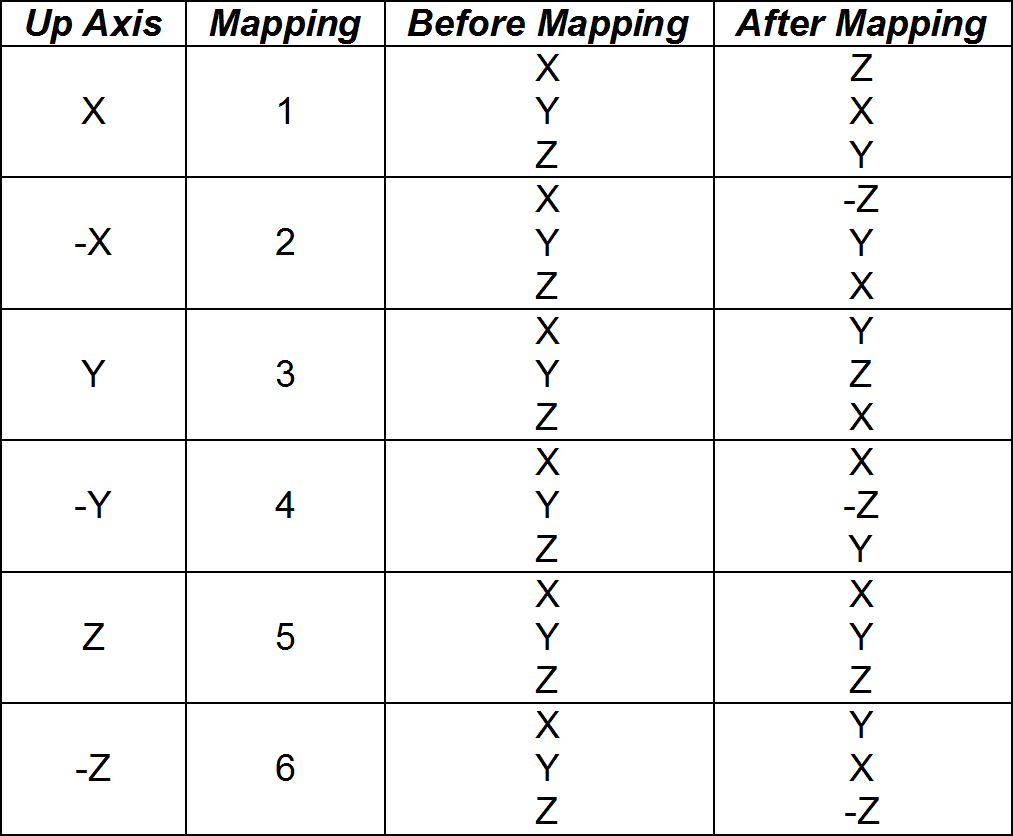Figure 3: Mapping 6 (Light is Vehicle, Dark is IMU)

Apply the effect of the mapping to the IMU axes, the result is a new set of IMU axes.

Starting with the vehicle axes (Y forward, X right, Z up), rotate around the vehicle Z axis until the vehicle Y axis is in the plane that the IMU Y axis would sweep out if rotated about its X axis (this is your Z input angle).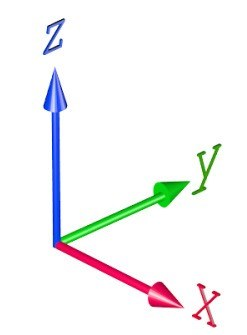Z angle

Now rotate around the vehicle X axis until the Y axes match (this is your X input angle).

X angle

Now rotate around the vehicle Y axis until both the X and Z axes match (this is your Y input angle).

Y angle

###### Example 1 (SPAN):

VEHICLEBODYROTATION 0 0 -90 SETIMUORIENTATION 6

In this example, the IMU is mounted with its Y axis backwards, X axis to the right and Z axis down. The vehicle frame is Y axis forward, X axis right and Z axis up.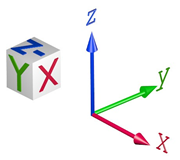Determine the IMU mapping:

IMU Mapping = 6 (Z down)Apply the mapping: Using the mapping (6) apply it to the IMU, the result is a new objective frame. This frame is also called the “computation” frame.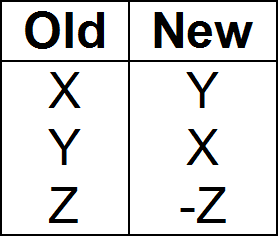New IMU FrameThe objective is to rotate from the vehicle frame to the new objective frame.

Rotate about Z: Starting from the vehicle frame, rotate first about the Z axis (solid blue)

Z = -90 degrees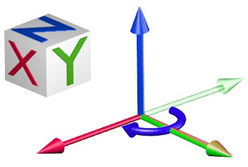Rotate about X: Because the Y axis now matches the IMU frame there is no need to rotate about X (solid red)

X = 0 degrees

Rotate about Y: Because X and Z already match the IMU frame there is no need to rotate about Y (solid green)

Y = 0 degrees

#### Input into SPAN:

SETIMUORIENTATION 6V EHICLEBODYROTATION 0 0 -90

###### Example 2 (SPAN):

VEHICLEBODYROTATION 0 0 -90 SETIMUORIENTATION 2

In this example, the IMU is mounted with its Z axis backwards, Y axis to the right and X axis down. The vehicle frame is Y axis forward, X axis right and Z axis up.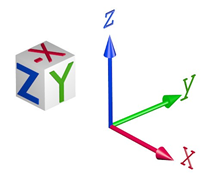Determine the IMU mapping:

IMU Mapping = 2 (X down)Apply the mapping: Using the mapping (2) apply it to the IMU, the result is a new objective frame. This frame is also called the “computation” frame.

New IMU Frame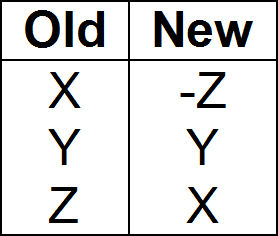The objective is to rotate from the vehicle frame to the new objective frame.

Rotate about Z: Starting from the vehicle frame, rotate first about the Z axis (solid blue)

Z = -90 degreesRotate about X: Because the Y axis now matches the IMU frame there is no need to rotate about X (solid red)

X = 0 degrees

Rotate about Y: Because X and Z already match the IMU frame there is no need to rotate about Y (solid green)

Y = 0 degrees

#### Input into SPAN:

SETIMUORIENTATION 2 VEHICLEBODYROTATION 0 0 -90